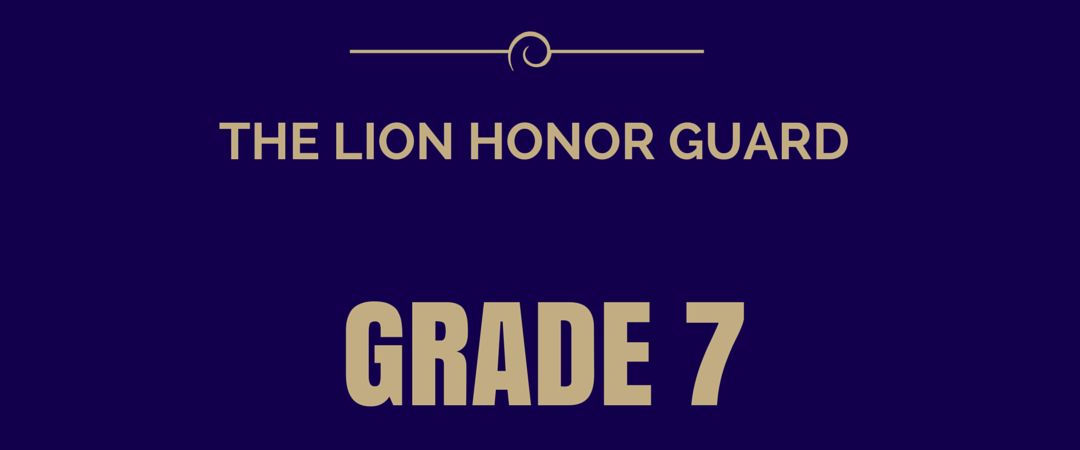Your student will be asked to delve deeply into a text, describe plot developments, character development, and support analysis with textual citations. Further, your student will learn the difference between the several different types of poetry and drama, including how they convey their central theme. In that vein, students will be asked to compare and contrast two works which deal with similar topics differently.

• read closely to determine what the text says explicitly and make logical inferences from it; cite specific textual evidence when writing or speaking to support conclusions drawn from the text;
• determine central ideas or themes of a text and analyze their development; summarize the key supporting details and ideas;
• analyze how and why individuals, events, and ideas develop and interact over the course of a text;
• describe how story elements influence the characters as the plot moves towards resolution;
• analyze the structure of texts, including how specific sentences, paragraphs and larger portions of the text relate to each other and the whole;
• understand and analyze how text structure contributes to the meaning of a drama or poem;
• understand and explain the development of the narrator’s or speaker’s point of view; and
• analyze how two or more texts address similar themes or topics in order to build knowledge or compare the approaches the authors take.

COMMON CORE EXPECTATIONS

• ability to identify and cite explicit textual evidence;
• draw inferences, supporting them with textual evidence;
• recognize and analyze theme development;
• write an objective summary;
• describe how characters change throughout a story or drama;
• interpret words and phrases as they are used in a text;
• understand connotations/denotations;
• understand figurative language;
• identify repetition of sounds;
• understand how word choice impacts meaning and tone;
• understand and identify meaning, types and structure of drama/poetry;
• assess how point of view or purpose shapes the content and style of a text;
• identify and contrast different points of view;
• integrate and evalutate diverse media and formats; and
• understand the difference between historical fiction and historical accounts.

In Grade 7, your student will focus on proportions; operations with rational numbers, expressions and linear equations; solve problems involving scale drawings and informal geometric constructions, work with two- and three-dimensional shapes; and draw inferences about populations based on population samples.

• analyze proportions and use them to solve real-world and mathematical problems;
• apply and extend previous understandings of operations with fractions to add, subtract, multiply, and divide rational numbers;
• use properties of operations to generate equivalent expressions;
• solve real-life and mathematical problems using numerical and algebraic expressions and equations;
• draw, construct and describe geometrical figures and describe the relationships between them;
• solve real-life and mathematical problems involving angle measure, area, surface area, and volume;
• use random sampling to draw inferences about a population;
• compare two populations; and
• work with probability models.

COMMON CORE EXPECTATIONS

• describe proportional relationships and use them to solve real-world problems;
• show an understanding of operations with fractions to add, subtract, multiply, and divide rational numbers;
• use properties of operations to generate equivalent expressions;
• solve real-life and mathematical problems using numerical and algebraic expressions and equations;
• draw, construct, and describe geometrical figures and describe the relationship between them;
• solve real-life and mathematical problems involving angle measure, area, surface area, and volume;
• use random sampling to draw conclusions about a population;
• draw informal comparative inferences about two populations; and
• investigate chance processes and develop, use, and evaluate probability models.